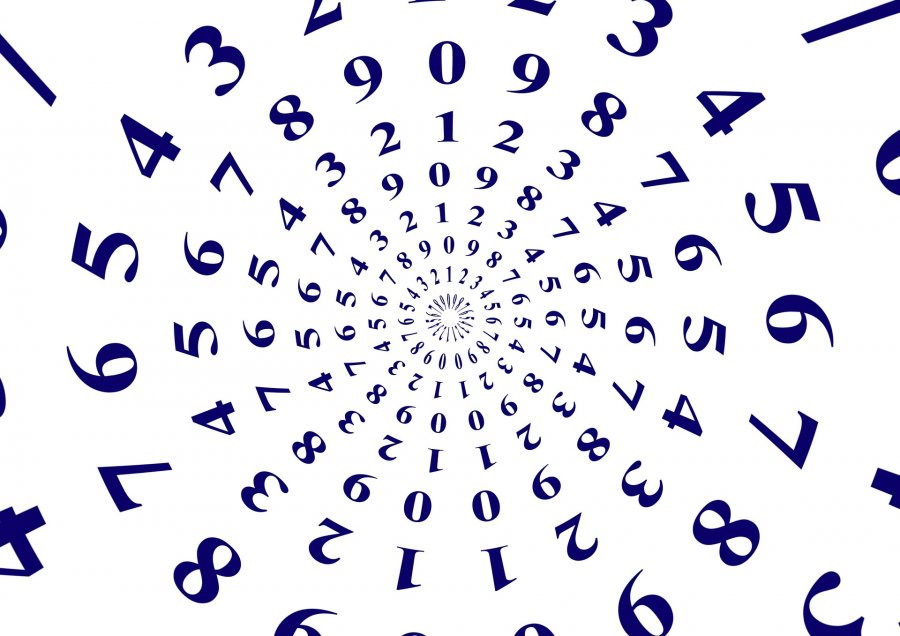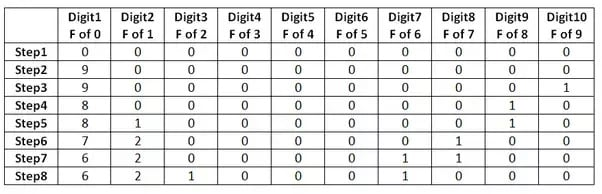# Finding secret number puzzle

409.5K Views

Find a secret 10-digit number where the first digit is equal to number of zeros in the number, the second digit is equal to number of one in the number and so on, until the tenth digit which is equal to number of nines in the number.Explanation-
Here is how I did it:The first digit = frequency of occurrence of the number 0
The Second digit = frequency of occurrence of the number 1(this was the tricky part)
.
.
.
The 10th digit = frequency of occurrence of the number 9.

I have written the digits in columns and started out with the number 0000000000 in step 1.

Step2: Since we have 10 zeroes, it means that the first digit of our number must be 10. But since 10 is not a digit, I will use 9. This also makes the number of zeroes in my number as 9.

Step3: Since the first digit is 9, it means that the 10th digit must be one (because it gives the frequency of 9 in our number.

Step4: But now we only have 8 zeroes in our number. So I will make the first digit as 8 and the 9th digit as 1.

Step5:Since my number has the digit 1, my second digit must be 1. But now I am left with only 7 zeroes.

Step6: I will make my first digit 7 (because after step5 I am left with only 7 zeroes). And since Step5 had 2 ones, I will make my second digit 2. OMG… but this now makes number of 1’s in my number 1 and not 2.  This means that I f the digit 1 is there in any place other than second digit, I am bound to come across this problem. So let me keep the second digit 2 and try to introduce another ‘1’ in some place.

Step7: Since I have to introduce one more 1, it means that the number of zeroes in my number have come down to 6. So my first digit will be six, my second digit will be 2 and my 6th digit will be 1.

Step8: The digit ‘1’ seems to be in the wrong place in Step 6. There is no ‘7’ but there is a 2. Let me just shift this ‘1’ from digit 8 to digit 3.

And there it is…6210001000